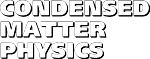Condensed Matter Physics, 2016, vol. 19, No. 3, 33703
DOI:10.5488/CMP.19.33703           arXiv:1503.06804

Title: Counting Majorana bound states using complex momenta
Author(s):
 I. Mandal (Perimeter Institute for Theoretical Physics, 31 Caroline St. N., Waterloo ON N2L 2Y5, Canada)

Recently, the connection between Majorana fermions bound to the defects in arbitrary dimensions, and complex momentum roots of the vanishing determinant of the corresponding bulk Bogoliubov–de Gennes (BdG) Hamiltonian, has been established (EPL, 2015, 110, 67005). Based on this understanding, a formula has been proposed to count the number (n) of the zero energy Majorana bound states, which is related to the topological phase of the system. In this paper, we provide a proof of the counting formula and we apply this formula to a variety of 1d and 2d models belonging to the classes BDI, DIII and D. We show that we can successfully chart out the topological phase diagrams. Studying these examples also enables us to explicitly observe the correspondence between these complex momentum solutions in the Fourier space, and the localized Majorana fermion wavefunctions in the position space. Finally, we corroborate the fact that for systems with a chiral symmetry, these solutions are the so-called "exceptional points", where two or more eigenvalues of the complexified Hamiltonian coalesce.

Key words: exceptional points, Majorana fermions, BDI, DIII, D, counting
PACS: 73.20.-r, 74.78.Na, 03.65.Vf

 Full text [pdf] << List of papers